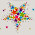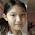## Wednesday, 2 July 2014

### Homework: Study Notes (p30) Discussion 2

Describe the various ways that we can use to find out if 3 given points lie on the same straight line.

Remember to enter your group number.

1.We can calculate the gradient of two points, thus we have to do this 3 times. If the gradient is the same and the y-intercept is the same as the previous point, the three points are in the same line

2.1. Calculate the gradient of the points and see if they are the same.
2. Calculate the y-intercept of the points and see if they are the same.
3. Draw a graph and see if they all fit on a same straight line.
(Group 1)

3.We can calculate the gradient of all the 3 different points,2 at a time,and if they are the same,1 1/2 in this case,the points lie on the same straight line.
Group 3

4.Calculate the gradient of the 3 points and the y-intercept see if it matches. If it is not, it means they are not on the same line

Kai Heng Group 3

5.Since it is a straight line, we can take any two points and calculate gradient using y1-y2/x1-x2.
If we have the equation, we can check with the gradient number since it is y=mx+c and m is gradient.
If we don't we can draw a graph and see if they all fit

Group 4 :)

6.We can find the gradient of every pair of points and if all three gradients are the same, they lie on the same line.

7.Draw the graph and mark out the three points, If the points are not in a straight line, then the three points are not on the same straight line.

8.1) Calculate the gradient of the first and second point.
2) Calculate the gradient of the second and third point.
3) If the gradient of both is the same, they are lying on the same line.

9.draw the graph out and see if the points are on the line
group four

10.1. Draw a graph and plot the points and use a ruler to see if all three can form a straight line.
2. Or find the gradient of any 2 points and find out the gradient of 2 other points(1point can be repeated). If they are all the same and have the same Y-intercept they will lie along the same line just at different places.

1.11.The three dots are on the same line cause their gradient is the same(gp3)

12.Join the first and second points by a line, and the second and third points by a line. If the equation of both lines are the same, then the 3 points lie on the same line.